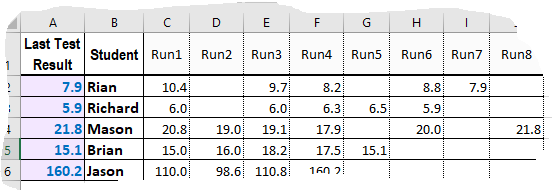## 10 July, 2021

### Complex Array formula

An array is a row or column of values, or a combination of them. Array formulas can be very useful in many applications where normal Excel formulas don't work. An array formula is a formula that can perform multiple calculations on one or more items in an array.

Array formulas can return either multiple results, or a single result. We can use array formulas to perform complex tasks, such as:

• create quickly sample datasets
• count some values contained in a range of cells
• sum numbers that meet certain conditions, such as the lowest values in a range
• sum every Nth value in a range of cells
• find specific values in a cell or a range of cells
• etc.

The following example shows you how the single-cell array formula can find the last filled cell in a given row and show its value. Here is the formula (all entered in a single cell):

And here's its application. I've entered it in cell A2 as array formula (using Ctrl+Shift+Enter) and copied it down the column A in order to automatically see values of the last filled cell in each of the rows, as illustrated below.The range used in the example formula (C:NA) can, obviously, be changed to fit a specific data set you're working on. This is just one example of using array formulas where regular formulas cannot provide desired results.